# NanoBlog Blog Actions

## Analog IC Design Course 7 nm FinFET Public Model Parameter Investigation

morreale Tuesday 09 of November, 2021

## 7 nm Model Parameters

Since the frequency response in homework 2 produced a unity gain frequency for the shorter gate length that wasn't higher than the long gate length FinFET, I suspect a problem with the model or the way I'm using it so I investigated the model further. The following model parameters were used for this investigation and for homework 3.

 parameter Model Value units Fin Length L 20.0 nm Number of fins NFIN 3 Number of fingers NFGR 1 Fin Height HFIN 32.0 nm Fin Thickness TFIN 6.5 nm Fin Pitch FPITCH 27.0 nm Fin Width, 1 fins W 70.5 nm Fin Width, 3 fins 211.5 nm W/L for 3 fins 10.6 Gate oxide thickness EOT 1 nm

The fin width was computed as NFIN (2 HFIN + TFIN) where HFIN is the fin height and TFIN is the fin thickness using the .model variables. This makes W/L = 10.6 for a FinFet with 3 fins.

## Extracted 7 nm lvt Model Parameters by Simulation

Using methods described in the course and in the book, the performance parameters for the 7 nm models were extracted. I haven't found a references to confirm the performance values yet.

 Parameter NMOS PMOS Units μCOX 156.6 110.2 μA/V2 Vt 230 -210 mV λ 0.1795 0.3563 1/V λL 0.0036 0.0071 μm/V COX fF/μm2 n 1.05 1.02 θ 2.94 1.92 V COV/W = LOVCOX 0.16 0.16 fF/μm Cdb/W ≈ CSb 5.5*10-8 5.6*10-8 fF/μm

The 7 nm models from the PTM website  are define with the .model function and have more parameters defined compared to the modified (simplified) model provided for the course. These models use the BSIM-CMG  model. The PTM website indicates that the model process maximum supply voltage is 700 mV. The homework, however, specifies VDS = 800 mV in the problems so was used for the solutions. The solutions are based on the materials presented in the course and the book Analog Integrated Circuit Design .

The sections below show how the parameters were determined and how the SIMetrix simulator was setup to make the measurements. Mathematica was used to add the line guides to estimate the projection of the gm slopes to the x-axis and to the projections of maximum gm.

### NMOS μnCOX, Vt, subthreshold slope, n, and θ

The schematic used for finding μnCOX, Vt, subthreshold slope, n, and θ is shown below.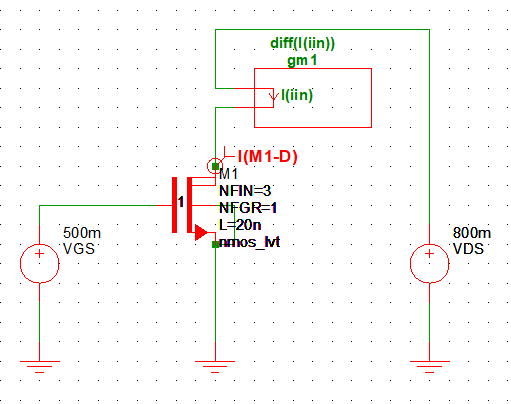The top plot in the figure below shows gm/ID vs VGS. The minimum was found to be 62.4 mV/decade using Measure -> 8 Minimum. n=1.05 was found using Ln(10)(nKT/q) = subthreshold slope (i.e n = 62.4 mV/(2.3kT/q)).

The middle plot shows gm vs VGS. The linear slope of gm is μnCOX(W/L) and was used to determine μnCOX at 156.6 μA/V2 for W/L = 211.5/20. The projection of the slope to the x-axis gives Vt at 230 mV. The voltage VGS at the intersection of where the projection of the gm slope to a line that is parallel to where gm flattens is used to determine Veff. At that point Veff = 1/(2θ). Solving for θ, θ = 2.9/V .

The bottom plot shows ID in the active region.### PMOS μpCOX, Vt, subthreshold slope, n, and θ

The schematic used for finding μpCOX, Vt, subthreshold slope, n, and θ is shown below.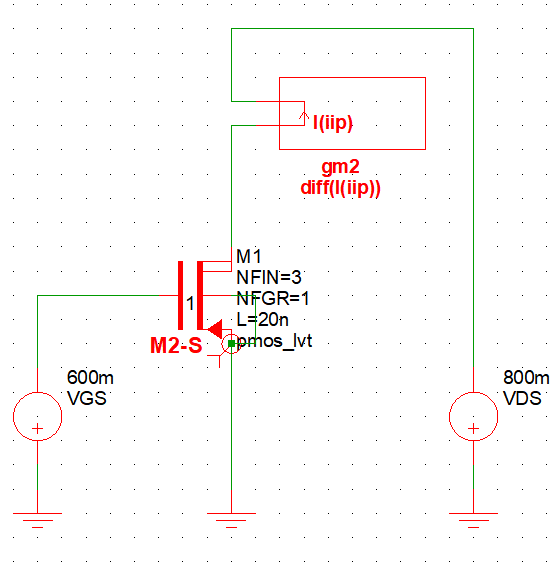The top plot in the figure below shows gm/ID vs VGS. The minimum was found to be 60.8 mV/decade using Measure -> 8 Minimum. n=1.02 was found using Ln(10)(nKT/q) = subthreshold slope (i.e n = 60.8 mV/(2.3kT/q)).

The middle plot shows gm vs VGS. The linear slope of gm is μpCOX(W/L) and was used to determine μpCOX at 110.2 μA/V2 for W/L = 211.5/20. The projection of the slope to the x-axis gives Vt at 210 mV. The voltage VGS at the intersection of where the projection of the gm slope to a line that is parallel to where gm flattens is used to determine Veff. At that point Veff = 1/(2θ). Solving for θ, θ = 1.9/V

The bottom plot shows ID in the active region.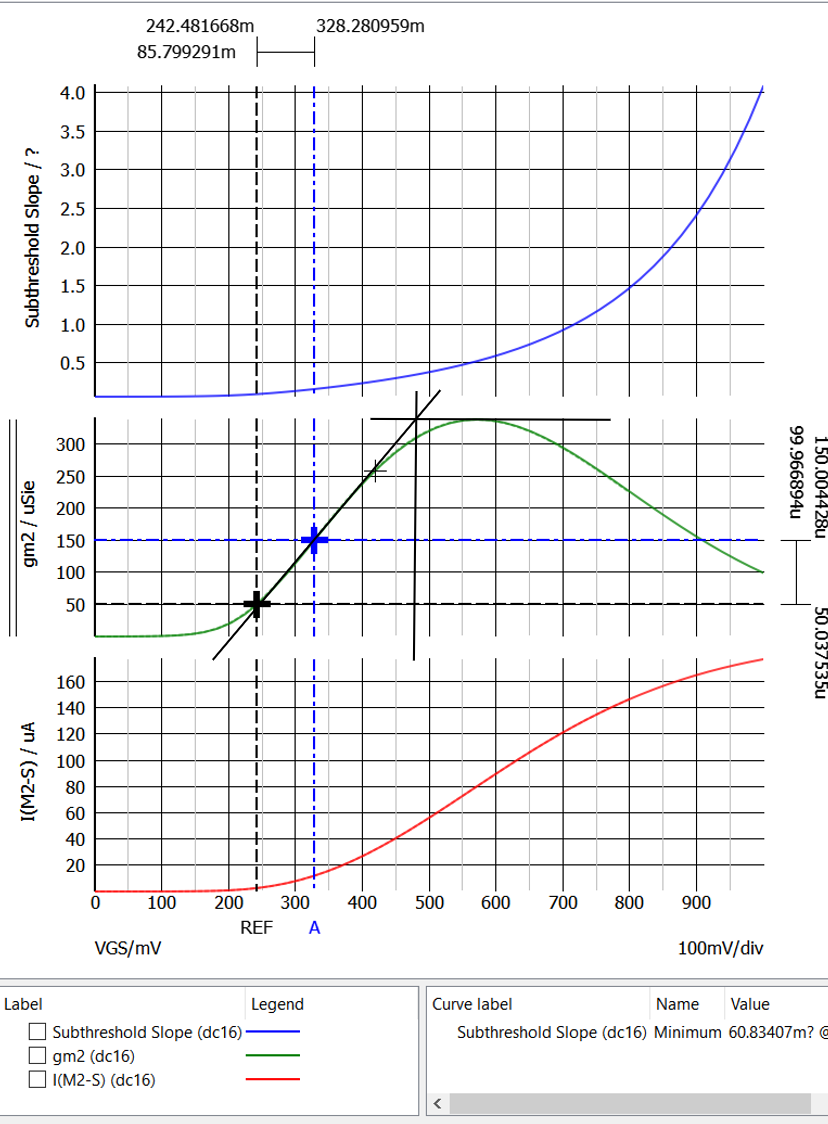### NMOS λL

The schematic below was used determine λ.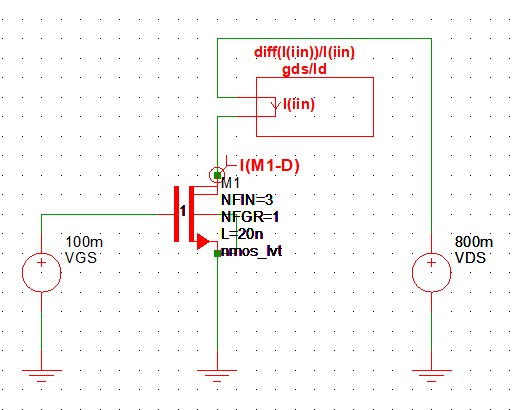The top plot in the figure below shows gds/ID and the bottom shows ID vs VDS for VGS = 300 mV, 500 mV, 800 mV, and 1 V. To generate a useful plot gds/ID, the Choose Analysis VDS sweep can't start a 0 V so that gds/ID doesn't become infinite.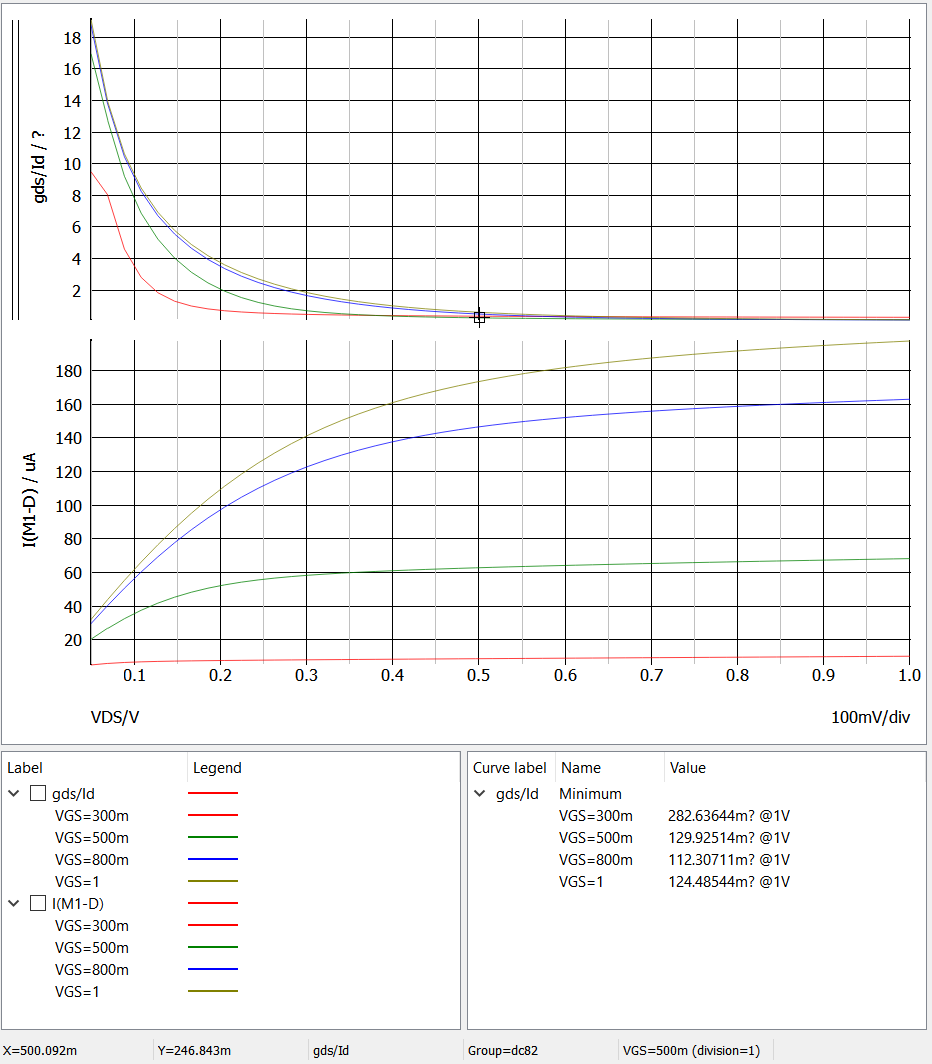gds/ID and ID vs VDS were plotted again but only for VGS = 500 mV to determine λ and λL. Recall that
$$g_{ds}=\frac{\partial I_d}{\partial V_{DS}}=\lambda (\frac{\mu_n C_{OX}}{2})(\frac{W}{L})V_{eff}^2=\lambda I_{D-sat}=\lambda I_d$$
In short, gds/ID = λ and was sampled in the middle of the active range at VDS = 654 mV as the start of the active region occurs at VDS-sat = Veff = 500 mV - 230 mV = 270 mV. λ = 0.1795/V and λL = 0.00359 μm/V .### PMOS λL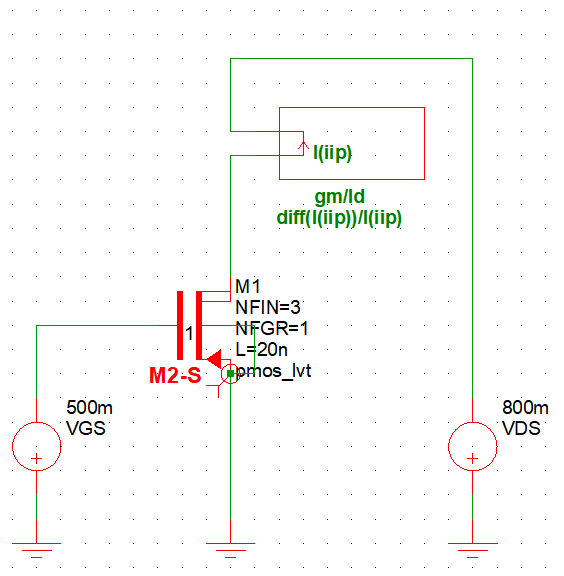gds/ID and ID vs VDS were plotted again but only for VGS = 500 mV to determine λ and λL. Recall that $$g_{ds}=\frac{\partial I_d}{\partial V_{DS}}=\lambda (\frac{\mu_p C_{OX}}{2})(\frac{W}{L})V_{eff}^2=\lambda I_{D-sat}=\lambda I_d$$
In short, gds/ID = λ and was sampled in the middle of the active range at VDS = 693 mV as the start of the active region occurs at VDS-sat = Veff = 500 mV - 210 mV = 290 mV. λ = 0.3563/V and λL = 0.00713 μm/V.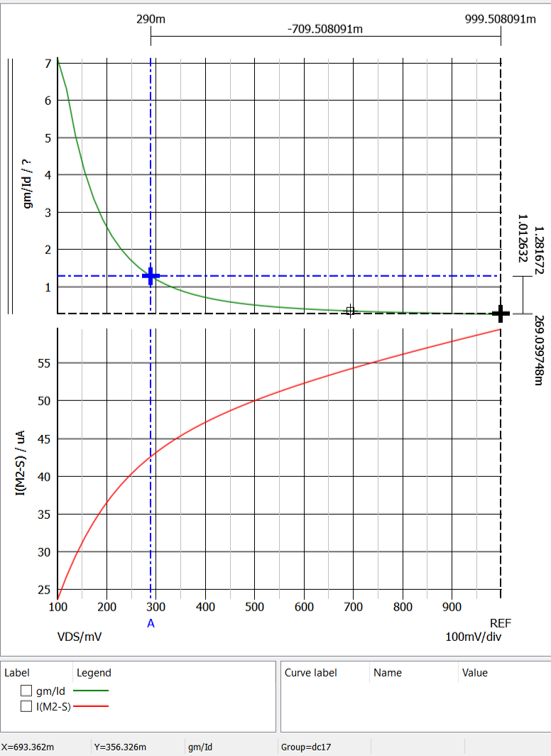### NMOS COV/W and Cdb/W

The schematic below was used to determine COV/W.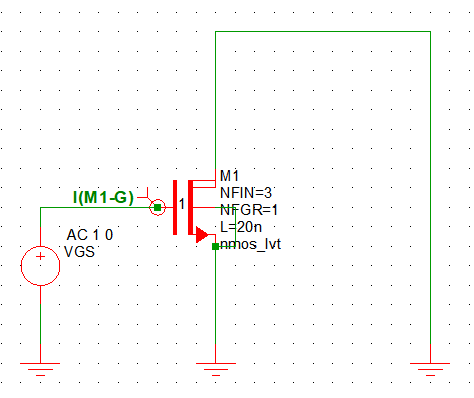The plot below shows the gate current Ig vs frequency. The gate current is function of frequency and Ig = 2πfVgCOV. Solving for COV, COV = Ig/(2πfVg) with f = 10 MHz and Vg = 2 V because the AC source produces a 1 V peak output. Ig = 4.25 nA at 10 MHz so COV/W = 0.16 fF/μm for the 211.5 nm gate width .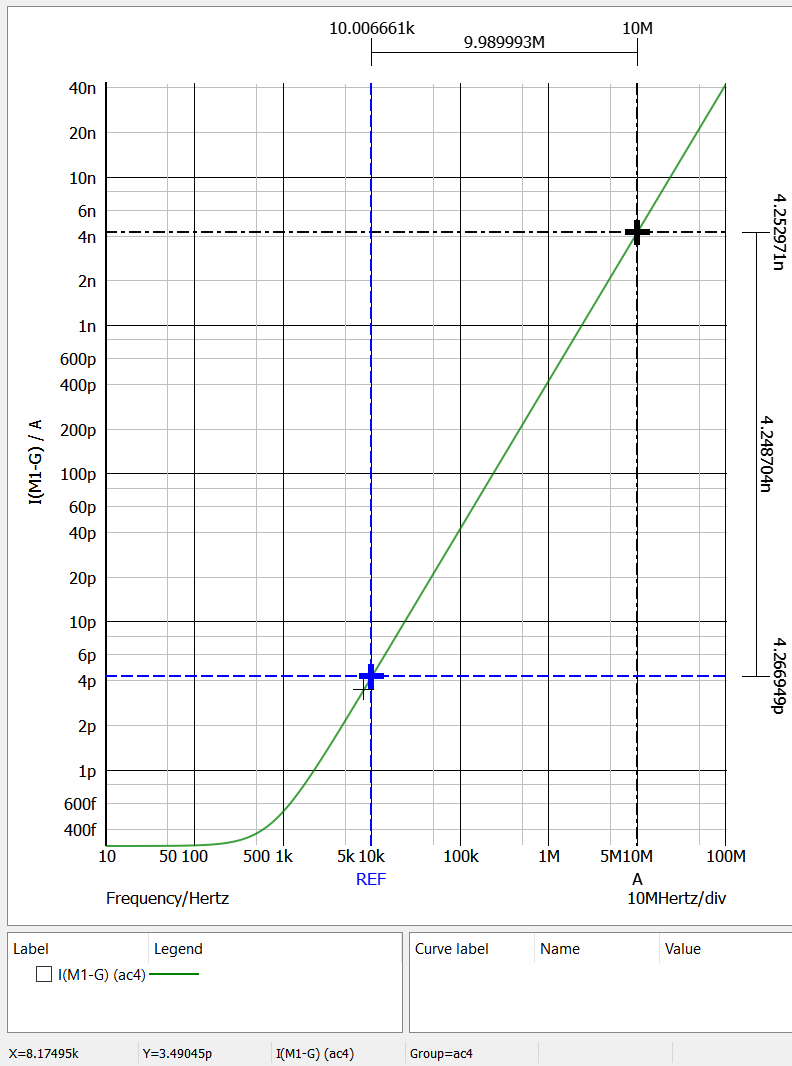The schematic below was used to find Cdb/W.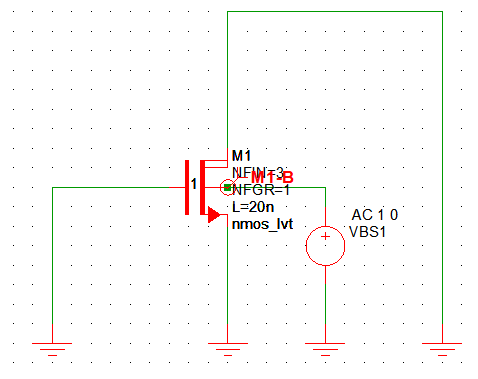The plot below shows the body current Ib vs frequency. The body current is function of frequency and Ib = 2πfVbCb where Cb = Cdb+Csb. Solving for Cb, Cb = Ib/(2πfVbs) with f = 10 MHz and Vb = 2 V because the AC source produces a 1 V peak output. Ib = 2.95 fA at 10 MHz so Cb/W = 1.1*10-7 fF/μm for the 211.5 nm gate width. For Cdb = Csb = Cb/2 = 5.5*10-8 fF/μm .### PMOS COV/W and Cdb/W

The schematic below was used to determine COV/W.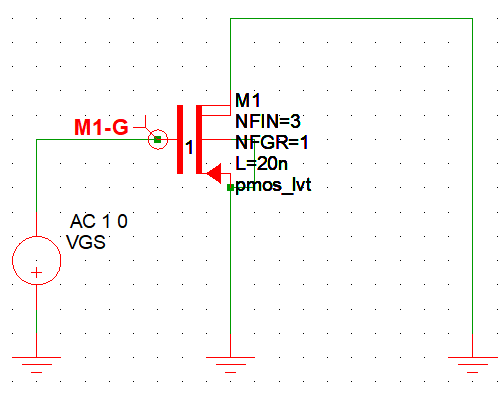The Simulate -> Choose Analysis setup for the AC analysis shown below.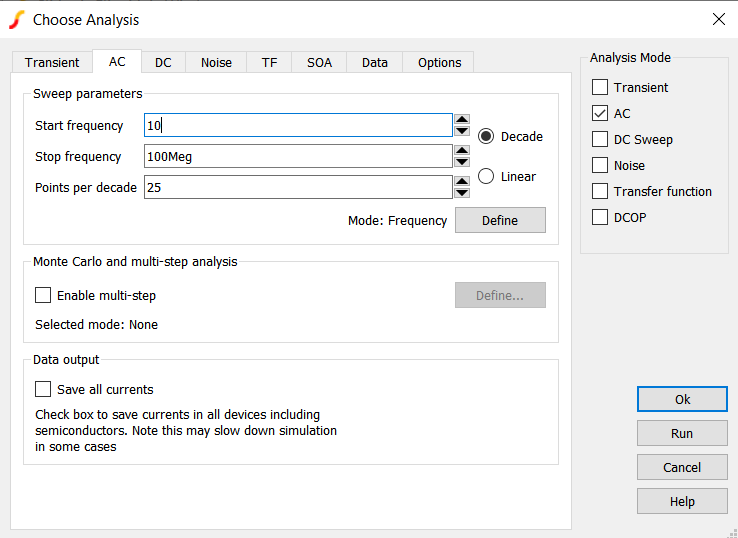Ig = 4.25 nA at 10 MHz so COV/W = 0.16 fF/μm for the 211.5 nm gate width.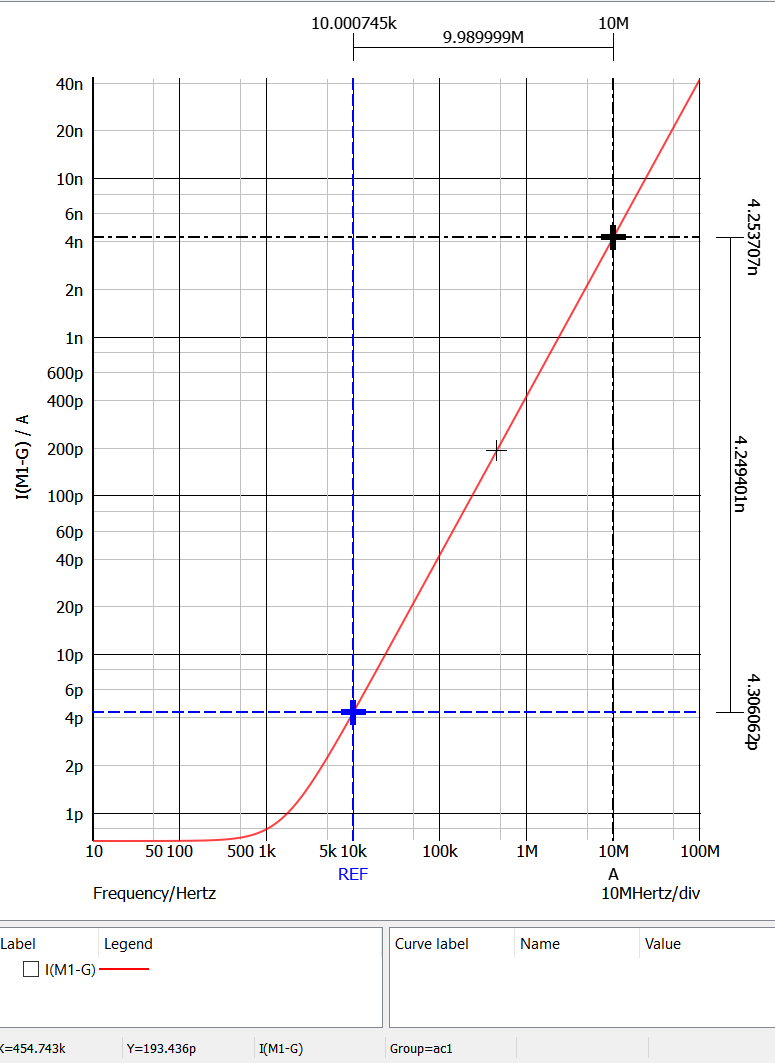The schematic below was used to find Cdb/W.Ib = 3.099 fA at 10 MHz so Cb/W = 1.13*10-7 fF/μm for the 211.5 nm gate width. For Cdb = Csb = Cb/2 = 5.57*10-8 fF/μm.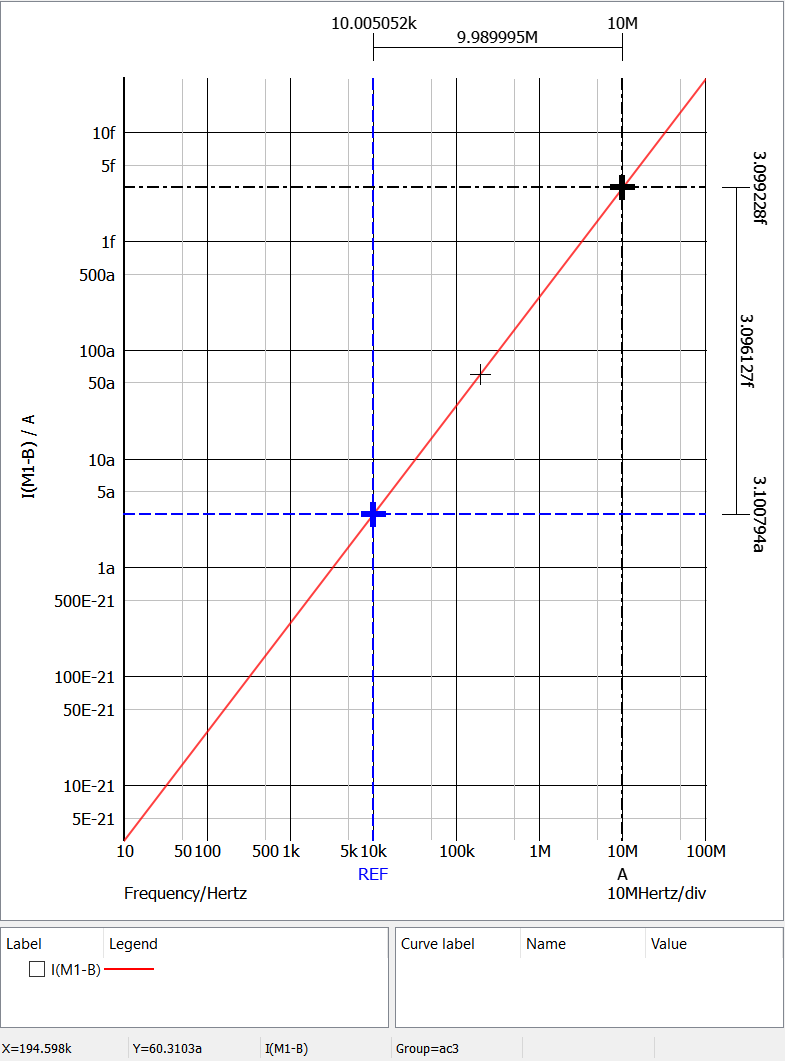### NMOS ID and Rin vs VGS

The gate and drain for the nmos_lvt and nmos_rvt transistors are connected together to simulated the IV curve of a diode connected transistor that is used as part of a current mirror. The MOSFET connected in this way isn't really a diode but its IV curve behaves like an diode IV curve because the VGS = VDS and the transistor operates in the active region. The ID vs VDS is

$$I_d=\frac{1}{2}\mu_n C_{OX} \frac{W}{L} (V_{GS}-V_t)^2 = \frac{1}{2} \mu_n C_{OX} \frac{W}{L}(V_{DS}-V_t)^2$$

Solving for VDS gives 

$$V_{DS}=\sqrt{\frac{I_d}{\frac{1}{2} \mu_n C_{OX} \frac{W}{L}}}+V_t$$

Using the model parameters in the table above at 100 μA, VDS = 577.5 mV for the nmos_lvt transistor, and VDS = 609.2 mV at 100 μA in the simulation. This represents a 32 mV error between the simulation and the calculation.

 Transistor VDS Simulation Units nmos_lvt 577.5 609.2 mV

The schematic below shows the diode connected nmos_lvt and nmos_rvt FinFETs in single and stacted configurations.The bottom plot in the figure below shows the ID vs VGS for the nmos_lvt and nmos-rvt transistors. The top two traces are the single gate-drain connected transistors, and the bottom two traces are the stacked transistors. The REF cursor is set at the ID = 100 μA for the single nmos_lvt transistor. The B cursor is set at the ID = 100 μA for the stacked nmos_lvt transistors. The middle plot shows gm for the transistors. The single transistors have the higher gm compared with the stacked transistors. The top plot shows the output resistance. The cursors was placed at 100 μA for the nmos_lvt device and the VDS is 609.2 mV and an resistance of 6.093 kOhms. The stacked nmos_lvt transistors have a VDS of 814.0 mV and a resistance of 8.138 kOhms.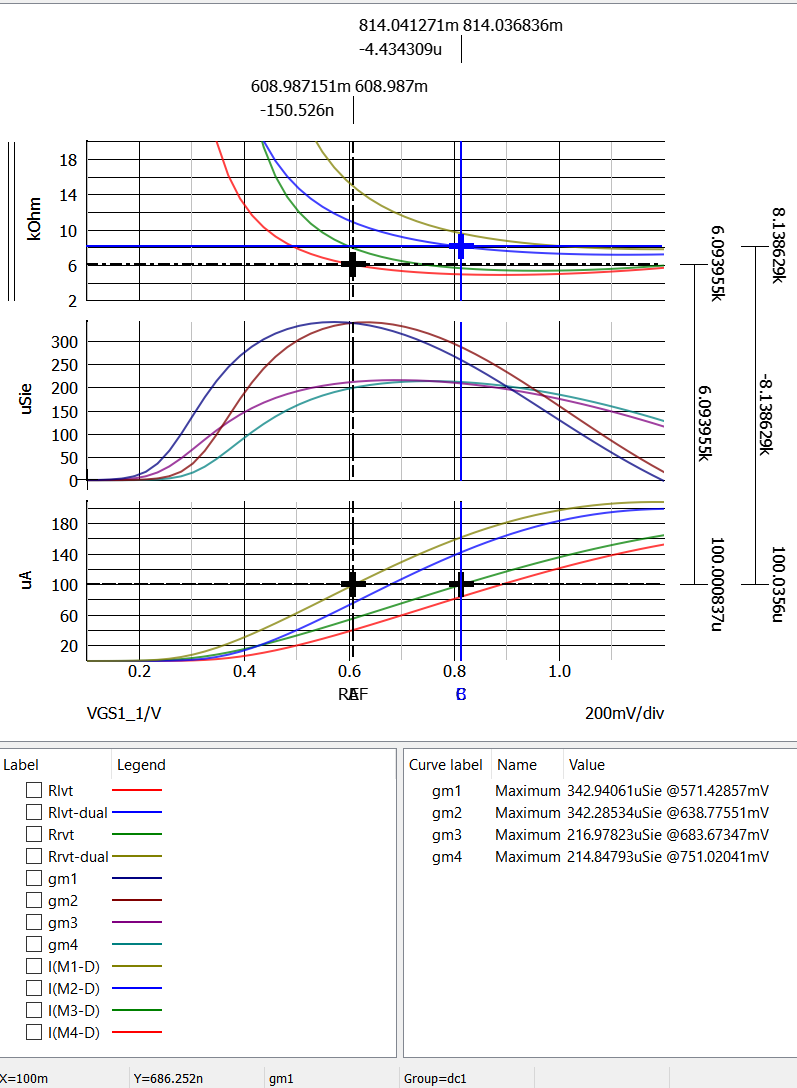### PMOS ID and Rin vs VGS

The simulation was repeated for the pmos_lvt and pmos_rvt transistors for both single and stacked configurations. The schematic for this simulation is shown below.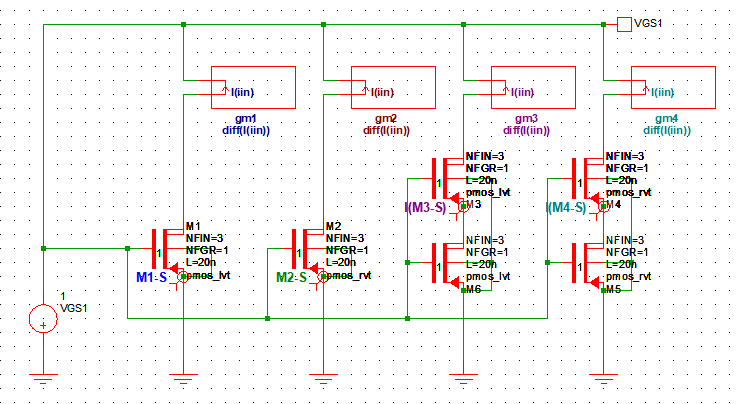The bottom plot in the figure below shows the ID vs VGS for the nmos_lvt and nmos-rvt transistors. The top two traces are the single gate-drain connected transistors, and the bottom two traces are the stacked transistors. The REF cursor is set at the ID = 100 μA for the single nmos_lvt transistor. The B cursor is set at the ID = 100 μA for the stacked nmos_lvt transistors. The middle plot shows gm for the transistors. The single transistors have the higher gm compared with the stacked transistors. The top plot shows the output resistance. The cursors was placed at 100 μA for the nmos_lvt device and the VDS is 650.5 mV and an resistance of 6.505 kOhms. The stacked nmos_lvt transistors have a VDS of 902.5 mV and a resistance of 9.021 kOhms.The figures below shows how the Choose Analysis and Define Curve configuration panels were setup to produce the plot above.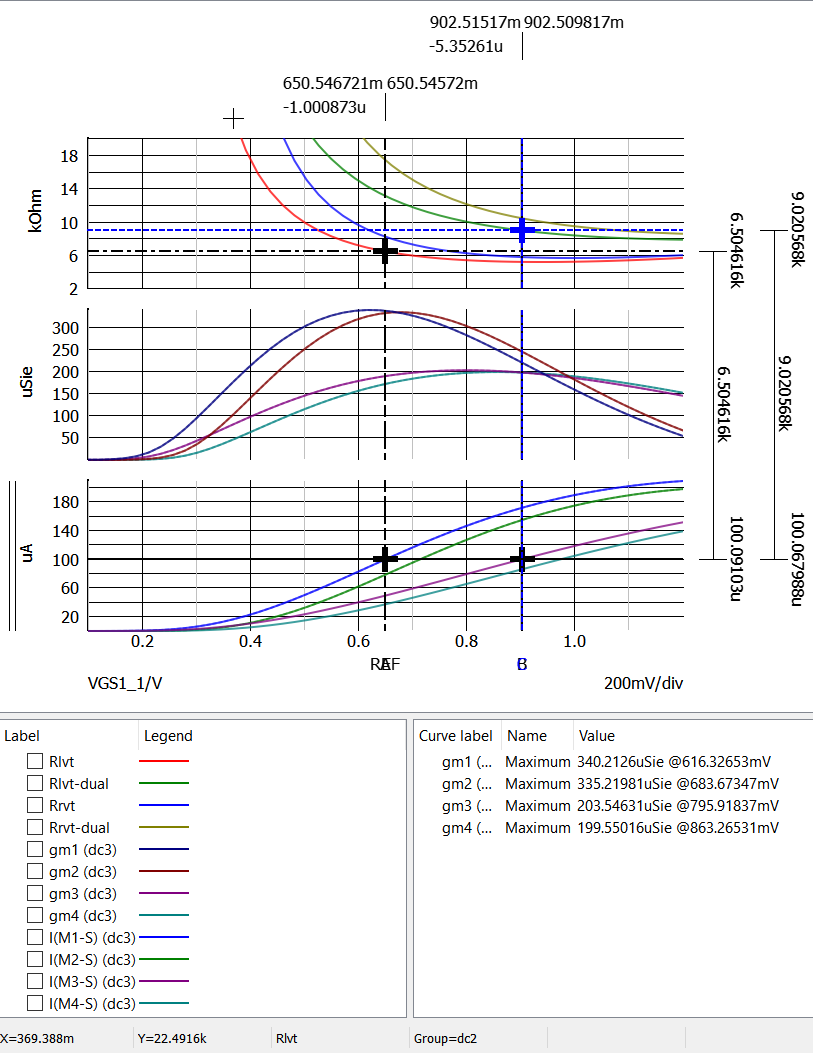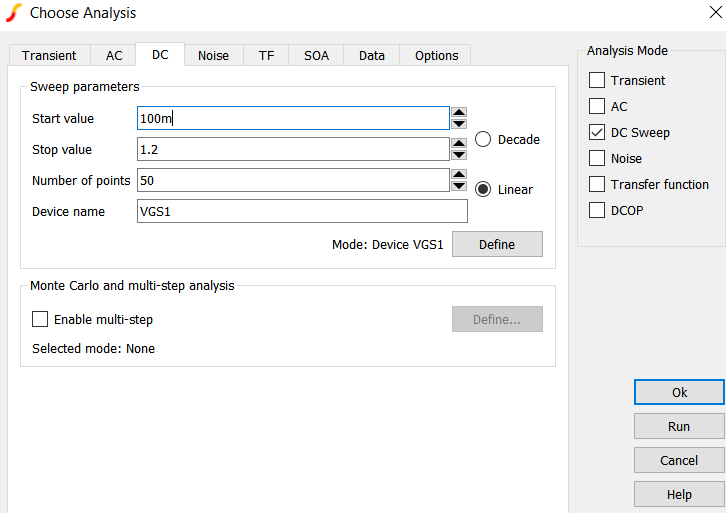## Index

: ASU Predictive Technology Model (PTM)
: BSIM Group Berkeley BSIM-CMG Model
: T. C. Carusone, D. A. Johns, and K. W. Martin, Analog Integrated Circuit Design, 2nd Edition. Wiley, 2011.
: T. C. Carusone, D. A. Johns, and K. W. Martin, Analog Integrated Circuit Design, 2nd Edition. Wiley, 2011. see pg. 28, 30, 47.
: T. C. Carusone, D. A. Johns, and K. W. Martin, Analog Integrated Circuit Design, 2nd Edition. Wiley, 2011. see pg. 29.
: 45nm CMOS process – Learning Microelectronics
: 45nm CMOS process – Learning Microelectronics.
: T. C. Carusone, D. A. Johns, and K. W. Martin, Analog Integrated Circuit Design, 2nd Edition. Wiley, 2011. see pg. 129.